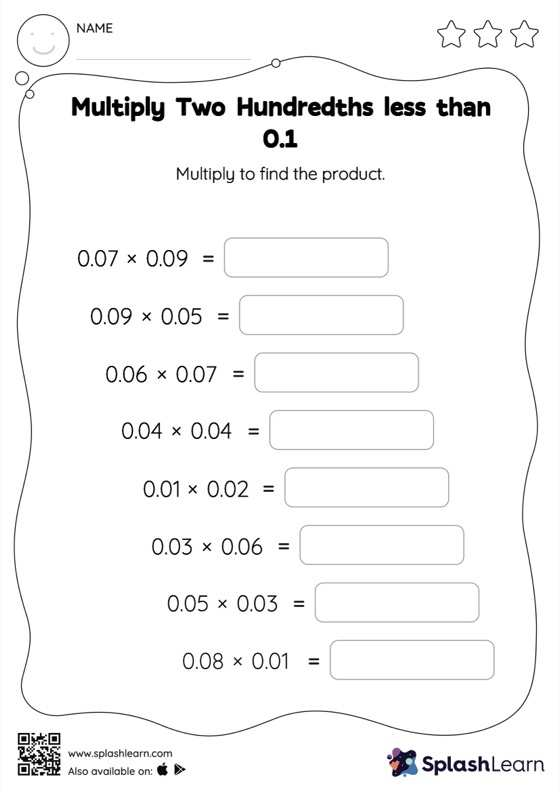# Multiply Two Hundredths less than 0.1: Horizontal Multiplication Worksheet

Home > Multiply Two Hundredths less than 0.1: Horizontal MultiplicationChildren use their math skills to complete this multiply two hundredths less than 0.1 Worksheet. When students multiply two decimals, they do so without considering the decimal point at first. After that, they count the decimal digits in each number and add the same number of digits to the right of the decimal point in the product. With the help of this multiply two hundredths less than 0.1 Worksheet, students can practice this idea in great depth.